# Cubes

Relevant articles: , , , , , , , .

[hide]

# Cubes

The$d$-cube or cube of dimension$d$, denoted by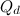$Q_d$, is the graph with vertex set consisting of all binary strings of length$d$ and with two vertices adjacent if and only if they differ in exactly one coordinate.

## Spectrum Results

Table 1 summarises the known results on the spectrum for the$d$-cube. An explanation of the sources of these results is given in .

Table 1$d$ Spectrum for the$d$-cube Possible exceptions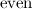${\rm even}$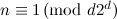$n \equiv 1 \,({\rm mod \ }d2^d)$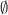$\emptyset$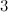$3$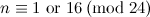$n \equiv 1 {\rm \ or \ } 16 \,({\rm mod \ }24)$$\emptyset$$5$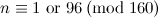$n \equiv 1 {\rm \ or \ } 96 \,({\rm mod \ }160)$$\emptyset$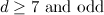$d\geq7{\rm \ and \ odd}$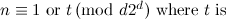$n \equiv 1 {\rm \ or \ } t \,({\rm mod \ }d2^d){\rm \ where \ }t{\rm \ is \ }$${\rm the \ unique \ integer \ satisfying}$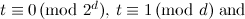$t\equiv 0 \,({\rm mod \ }2^d),\, t\equiv 1 \,({\rm mod \ }d){\rm \ and}$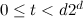$0\leq t$n\equiv t \,({\rm mod \ }d2^d){\rm \ with \ }n{\rm \ not }$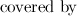${\rm covered \ by }$ Theorem 1,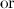${\rm or }$ Theorem 2,${\rm \ or \ }$

Theorem 1 

Let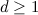$d \geq 1$ be odd, let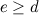$e \geq d$ be such that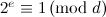$2^e \equiv 1\,({\rm mod \ }d)$ and let$s$ be the order of$2 \,({\rm mod \ }d)$. If$t$ is a non-negative integer and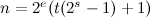$n=2^e(t(2^s-1)+1)$, then there exists a$d$-cube-design of order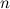$n$.

Theorem 2 

Let$d \geq 1$ be odd and let$e \geq d$ be such that$2^e \equiv 1\,({\rm mod \ }d)$. If$t$ is a non-negative integer and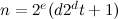$n=2^e(d2^dt+1)$, then there exists a$d$-cube-design of order$n$.

## Notes

• The$1$-cube is a single edge and the spectrum is trivially the set of all positive integers.
• The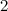$2$-cube is a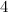$4$-cycle and the spectrum is well known to be all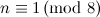$n\equiv 1\,({\rm mod \ }8)$ (see the section on cycles).

## References

1. 1.0 1.1 Adams, P., Bryant, D., and Buchanan, M. A survey on the existence of G-designs, J. Combin. Des. 16, 373–410 (2008).
2. Bryant, D. E., El-Zanati, S. I., and Gardner, R. B. Decompositions of K_m,n and K_n into cubes, Australas. J. Combin. 9, 285–290 (1994).
3. Bryant, D., El-Zanati, S. I., Maenhaut, B., and Vanden Eynden, C. Decomposition of complete graphs into 5-cubes, J. Combin. Des. 14, 159–166 (2006).
4. 4.0 4.1 Buratti, M. (private communication).
5. 5.0 5.1 El-Zanati, S. I. and Vanden Eynden, C. Decomposing complete graphs into cubes, Discuss. Math. Graph Theory, 26, 141–147 (2006).
6. Kotzig, A. Decompositions of complete graphs into isomorphic cubes, J. Combin. Theory Ser. B, 31, 292–296 (1981).
7. Maheo, M. Strongly graceful graphs, Discrete Math. 29, 39–46 (1980).
8. Wilson, R. M. Decompositions of complete graphs into subgraphs isomorphic to a given graph, Proceedings of the Fifth British Combinatorial Conference (Univ. Aberdeen, Aberdeen, 1975), Congressus Numerantium, No. XV, Utilitas Math., Winnipeg, Man. 647–659 (1976).